Test: Basic Concept of Rotating Electrical Machines of Electrical Machines

# Test: Basic Concept of Rotating Electrical Machines of Electrical Machines

Test Description

## 10 Questions MCQ Test GATE Electrical Engineering (EE) 2023 Mock Test Series | Test: Basic Concept of Rotating Electrical Machines of Electrical Machines

Test: Basic Concept of Rotating Electrical Machines of Electrical Machines for Electrical Engineering (EE) 2023 is part of GATE Electrical Engineering (EE) 2023 Mock Test Series preparation. The Test: Basic Concept of Rotating Electrical Machines of Electrical Machines questions and answers have been prepared according to the Electrical Engineering (EE) exam syllabus.The Test: Basic Concept of Rotating Electrical Machines of Electrical Machines MCQs are made for Electrical Engineering (EE) 2023 Exam. Find important definitions, questions, notes, meanings, examples, exercises, MCQs and online tests for Test: Basic Concept of Rotating Electrical Machines of Electrical Machines below.
Solutions of Test: Basic Concept of Rotating Electrical Machines of Electrical Machines questions in English are available as part of our GATE Electrical Engineering (EE) 2023 Mock Test Series for Electrical Engineering (EE) & Test: Basic Concept of Rotating Electrical Machines of Electrical Machines solutions in Hindi for GATE Electrical Engineering (EE) 2023 Mock Test Series course. Download more important topics, notes, lectures and mock test series for Electrical Engineering (EE) Exam by signing up for free. Attempt Test: Basic Concept of Rotating Electrical Machines of Electrical Machines | 10 questions in 30 minutes | Mock test for Electrical Engineering (EE) preparation | Free important questions MCQ to study GATE Electrical Engineering (EE) 2023 Mock Test Series for Electrical Engineering (EE) Exam | Download free PDF with solutions
 1 Crore+ students have signed up on EduRev. Have you?
Test: Basic Concept of Rotating Electrical Machines of Electrical Machines - Question 1

### A linear magnetic circuit has flux linkages of 1.2 Wb turns when a current of 10 A flows through its coil. The energy stored in the magnetic field of this coil, in Joules is

Detailed Solution for Test: Basic Concept of Rotating Electrical Machines of Electrical Machines - Question 1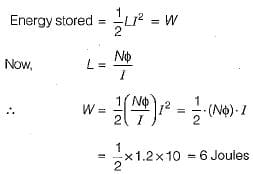Test: Basic Concept of Rotating Electrical Machines of Electrical Machines - Question 2

### The electromagnetic force and/or torque, developed in any physical system, acts in such a direction as to tend to

Test: Basic Concept of Rotating Electrical Machines of Electrical Machines - Question 3

### Singly-and doubly-excited magnetic systems are respectively

Detailed Solution for Test: Basic Concept of Rotating Electrical Machines of Electrical Machines - Question 3

In synchronous-motor, dc excitation is given to rotor for flux generation while it’s stator is connected is 3-φ supply. Therefore, it has doubly- excited system . Reluctance motor is an excitation-less motor ie. it has singly-excited magnetic system.

Test: Basic Concept of Rotating Electrical Machines of Electrical Machines - Question 4

A rotating electromechanical energy conversion device has uniform air-gap. If δ is the space angle between the axis of stator field and rotor field, then the average torque developed is proportional to (a and b are constants)

Detailed Solution for Test: Basic Concept of Rotating Electrical Machines of Electrical Machines - Question 4

Average torque developed is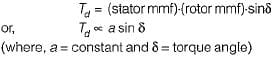Test: Basic Concept of Rotating Electrical Machines of Electrical Machines - Question 5

Armature winding is one in which working

Test: Basic Concept of Rotating Electrical Machines of Electrical Machines - Question 6

In ac rotating machines, the generated or speed emf

Detailed Solution for Test: Basic Concept of Rotating Electrical Machines of Electrical Machines - Question 6

In ac rotating machines like synchronous generators/motor/induction motors, working flux (φ) leads generated emf by 900. However, in static device like a transformer working flux (φ) lags the applied voltage by 900.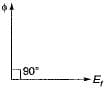Test: Basic Concept of Rotating Electrical Machines of Electrical Machines - Question 7

A 3-phase, 4-pole alternator has 48 stator slots carrying a 3-phase distributed winding. Each coil of the winding is short chorded by one slot pitch. The belt-factor of the winding is given by

Detailed Solution for Test: Basic Concept of Rotating Electrical Machines of Electrical Machines - Question 7

m = number of slots/pole /phase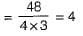Number of slots /pole = 48/4 = 12
∴ slot-pitch angle,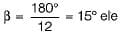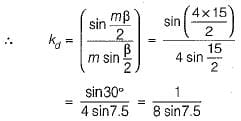Test: Basic Concept of Rotating Electrical Machines of Electrical Machines - Question 8

Consider the following statements about the chorded coils:
1. Chorded coil leads to reduction in harmonics.
2. In chorded coils, coil span may be less or more than 180° electrical.
3. Chorded coils require more conductors material.
4. Chorded coils reduce the magnitude of output voltage.
5. Chorded coils give better generated emf waveform.
6. In chorded coils, coil span is less than 180° electrical.
From these, the correct statements are:

Test: Basic Concept of Rotating Electrical Machines of Electrical Machines - Question 9

For eliminating 3rd harmonic from the phase emf generated in an alternator, the coil span in terms of full-pitch would be

Detailed Solution for Test: Basic Concept of Rotating Electrical Machines of Electrical Machines - Question 9

For eliminating nth harmonic,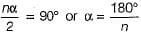∴ Coil span of short-pitched winding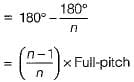For eliminating 3rd harmonic, coil-span
= 2/3 x Full-pitch

Test: Basic Concept of Rotating Electrical Machines of Electrical Machines - Question 10

If the dimensions of all the parts of a synchronous generator, and the number of field and armature turns are doubled, then the generated voltage will change by a factor of

Detailed Solution for Test: Basic Concept of Rotating Electrical Machines of Electrical Machines - Question 10

Emf induced per phase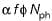Here,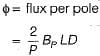( L = Axial length of armature and D = diameter)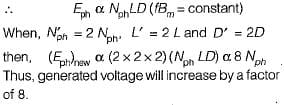## GATE Electrical Engineering (EE) 2023 Mock Test Series

22 docs|274 tests
Information about Test: Basic Concept of Rotating Electrical Machines of Electrical Machines Page
In this test you can find the Exam questions for Test: Basic Concept of Rotating Electrical Machines of Electrical Machines solved & explained in the simplest way possible. Besides giving Questions and answers for Test: Basic Concept of Rotating Electrical Machines of Electrical Machines, EduRev gives you an ample number of Online tests for practice

## GATE Electrical Engineering (EE) 2023 Mock Test Series

22 docs|274 tests(Scan QR code)